# 基于 Arduino Uno 的太阳能充电电池供电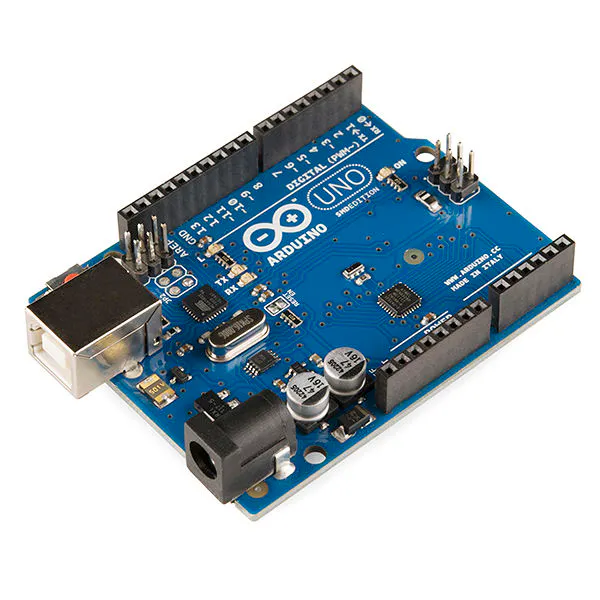• Arduino Uno
• 小面包板
• 5V 升压器
• 锂电池充电器（TP4056）
• 6V太阳能电池
• 18560锂电池
• 电池座
• 1N4004 二极管 (x2)
• 555集成电路
• 2N3904 三极管（x2）
• 1 兆欧电阻 (x2)
• 100 kohm 电阻器 (x3)
• 10 kohm 电阻 (x1)
• 100 uF 电解电容器 (x2)
• 10 nF 陶瓷电容器 (x1)
• 5V 单刀双掷继电器
• 跳线
• USB电缆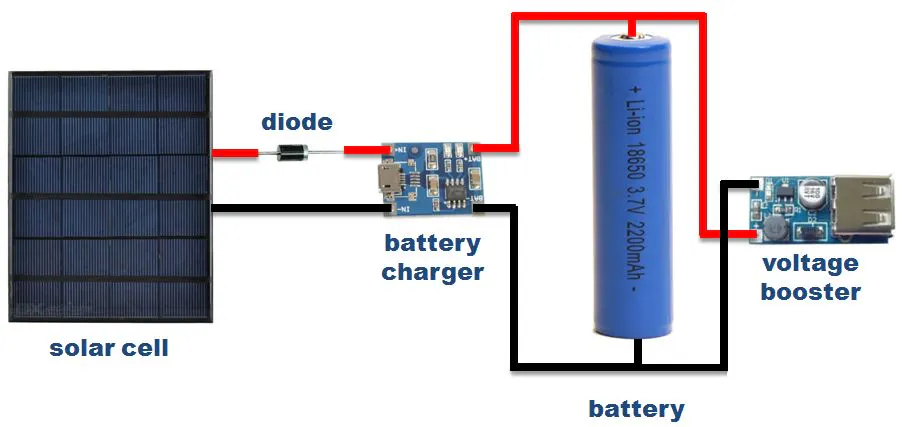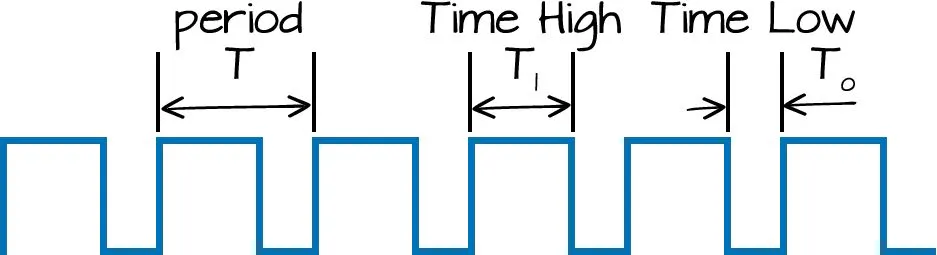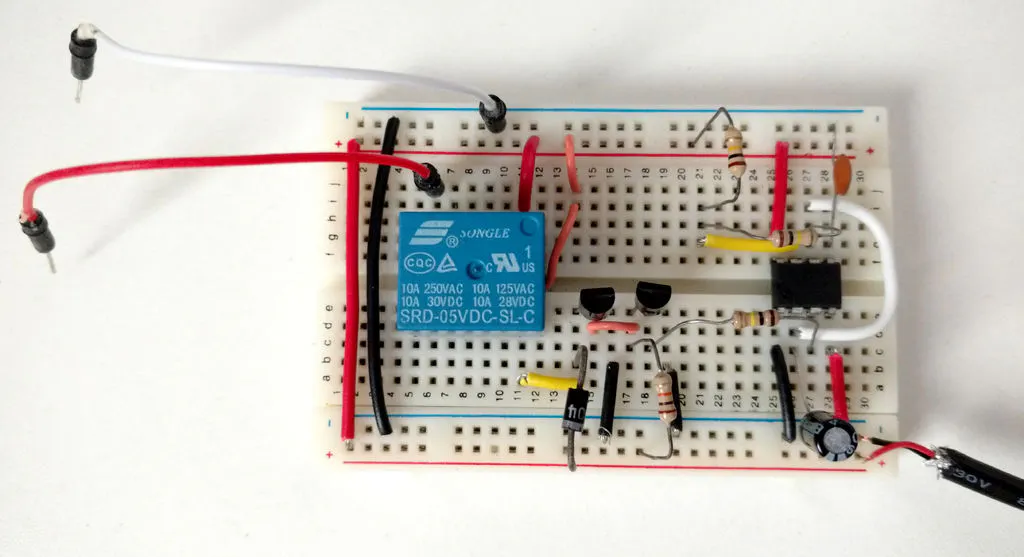• R1 = 2 兆欧
• R2 = 200 千欧
• R4 = 10 欧姆
• R5 = 10千欧姆
• C1 = 200 uF
• C2 = 10 nF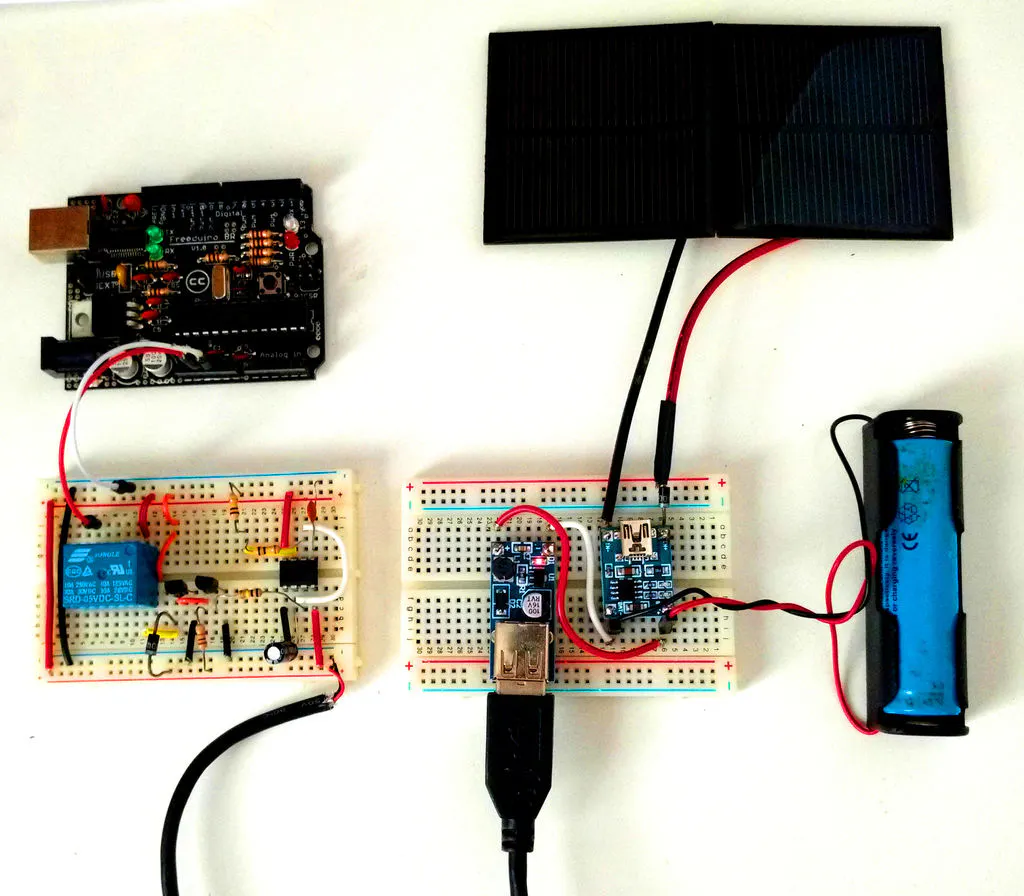Arduino 将每 5 分钟通电一次并保持 27 秒。您可以更改这些值，为之前描述的电阻器和电容器选择不同的值。

• 平均电流 (Iavg) = (Ton*Ion + Toff*Ioff ) / (Ton +Toff)
• 吨（Arduino 处于活动状态）= 27 秒
• 离子 = 51.7 mA
• Toff（arduino 关闭）= 5 分钟 = 300 秒
• Ioff = 34.9 毫安
• Iavg = 36.3 毫安
• 工作电压 (Vo) = 5V
• 平均功率 (Pavg) = Vo * Iavg = 5 * 36.3 = 181 mW
• 锂离子电池容量 = 3000 mAh
• 电池电压 = 3.7V
• 功率 =3.7 * 3000 = 11100 毫瓦时
• 电池寿命 = 11100/181 = 61 小时 = 2.5 天

• 平均电流 (Iavg) = (Ton*Ion + Toff*Ioff ) / (Ton +Toff)
• 吨（arduino 处于活动状态）= 27 s
• 离子 = 92 mA
• Toff（arduino 关闭）= 5 分钟 = 300 秒
• Ioff = 0.8 毫安
• Iavg = 8.2 毫安
• 工作电压 (Vo) = 5V
• 平均功率 (Pavg) = Vo * Iavg = 5 * 8.2 = 41 mW
• 锂离子电池容量 = 3000 mAh
• 电池电压 = 3.7V
• 功率 =3.7 * 3000 = 11100 毫瓦时
• 电池寿命 = 11100/41 = 270 小时 = 11 天加入微信技术交流群关注与非网服务号加入电路城 QQ 交流群